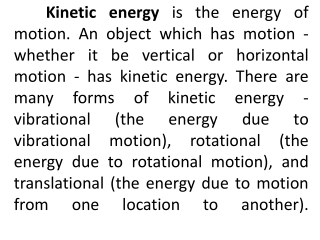DownloadDownload Presentationwhere m = mass of object v = speed of object

# where m = mass of object v = speed of object

Download Presentation## where m = mass of object v = speed of object

- - - - - - - - - - - - - - - - - - - - - - - - - - - E N D - - - - - - - - - - - - - - - - - - - - - - - - - - -
##### Presentation Transcript

1. Kinetic energy is the energy of motion. An object which has motion - whether it be vertical or horizontal motion - has kinetic energy. There are many forms of kinetic energy - vibrational (the energy due to vibrational motion), rotational (the energy due to rotational motion), and translational (the energy due to motion from one location to another).

2. To keep matters simple, we will focus upon translational kinetic energy. The amount of translational kinetic energy (from here on, the phrase kinetic energy will refer to translational kinetic energy) which an object has depends upon two variables: the mass (m) of the object and the speed (v) of the object. The following equation is used to represent the kinetic energy (KE) of an object.

3. where m = mass of objectv = speed of object This equation reveals that the kinetic energy of an object is directly proportional to the square of its speed. That means that for a twofold increase in speed, the kinetic energy will increase by a factor of four.

4. Thermal energy – is the total potential energy and kinetic energy of an object’s particle.Convection – is heat transfer movement of matter. When water is heated on a stove, for example, water near the bottom of the of the pot rises by convection.

5. Conduction – is the heat transfer by movement of particles. Example: A metal spoon in a bowl of hot soup is warmed by conduction of heat from particle to particle along the length of the spoon. Radiation – is the heat transfer by electromagnetic waves. Unlike convection and conduction, radiation can move through empty space as well as through matter. Example: energy from the sun travels by radiation through space.

6. The temperature of the substance increaess as it absorbs heat. Specific heat is the amount of heat needed to raise the temperature of one kilogram of a substance by 10C. Land near a lake or ocean, for example, changes temperature faster than water that is nearby because water has a higher specific heat than land.

7. Radiant Energy – energy coming from the sun. Mechanical Energy – energy of moving objects.

8. ENERGY CHANGES Energy often changes from one form to another. A rock on a cliff has only gravitational potential energy, but if the rock falls, the energy changes to kinetic energy. A light bulb changes electrical energy to thermal energy and light. Chemical energy in gasoline changes to kinetic energy as a car moves.